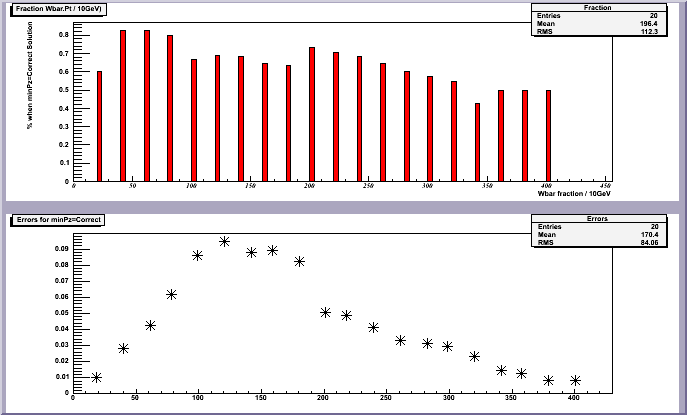# Put custom errors

Hello

I would like to ask this - I have in a histo some function. How can I put “custom” error bars on this plot, ie not using the draw(“E”) options but errors with calculated values out of another calculation for example?

use TH1::SetBinError

Rene

Yes, I already have done this but without result…

Look this first plot - top is the ratios, while bottom is my custom errors (1.png) - seperate plots, to see, that the errors are really small numbers

Now, 2.png shows the result after a loop for the calcutation and the custom SetBinError - it looks like (don’t mind if you don’t see entries after 250GeV, I just runned this for fewer events)

[code]{for int i=0;i<20;i++){

Fraction->Fill(i40, fraction[i]);
Fraction->SetBinError(i
40,binomial[i]);
}[/code]

After that, at the end, I do
Fraction->Draw();

It is obvious, if you compare 1.png with 2.pn, that the error bars are oversized, while I don’t even see the fraction bars from the 1.png plot…

Nevertheless, if I do Fraction->Draw(“E”); I get same exactly wrong plot…

Can you help me you with this?Could you send the shortest possible RUNNING script reproducing your case?
For your code extract, I have the feeling that you are setting the error for the wrong bin number :First bin is bin 1, underflow bin is bin 0.

Rene

```Fraction->Fill(i*40, fraction[i]); ```by
```Fraction->SetBinContent(i*40, fraction[i]); ```